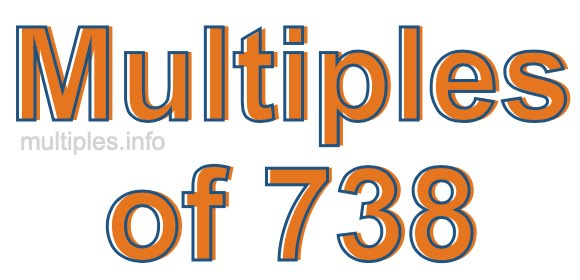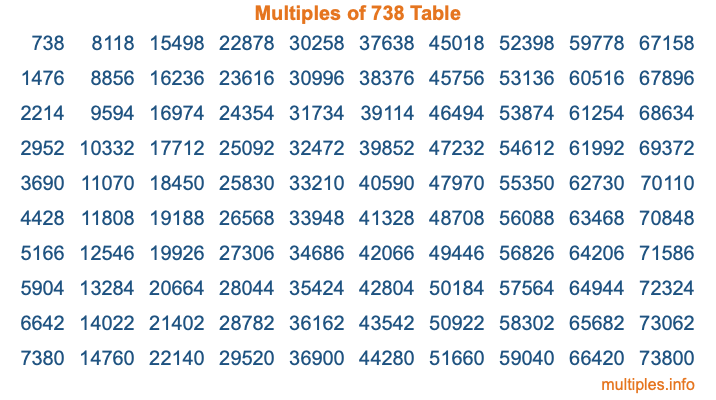Multiples of 738Welcome to the Multiples of 738 page. Here we will first teach you everything you will ever need to know about the multiples of 738, and then give you a study guide summary of everything we taught you to make sure you remember it all. Use this page to look up facts and learn information about the multiples of 738. This page will make you a multiples of seven hundred thirty-eight expert!

Definition of Multiples of 738
Multiples of 738 are all the numbers that when divided by 738 equal an integer. Each of the multiples of 738 are called a multiple. A multiple of 738 is created by multiplying 738 by an integer.

Therefore, to create a list of multiples of 738, you start with 1 multiplied by 738, then 2 multiplied by 738, then 3 multiplied by 738, and so on for as long as you want. Thus, the list of the first five multiples of 738 is 738, 1476, 2214, 2952, and 3690. To see a larger list of multiples of 738, see the printable image of Multiples of 738 further down on this page. We also have a category where you can choose any nth multiple of 738.

Multiples of 738 Checker
The Multiples of 738 Checker below checks to see if any number of your choice is a multiple of 738. In other words, it checks to see if there is any number (integer) that when multiplied by 738 will equal your number. To do that, we divide your number by 738. If the the quotient is an integer, then your number is a multiple of 738.

Is  a multiple of 738?

Least Common Multiple of 738 and ...
A Least Common Multiple (LCM) is the lowest multiple that two or more numbers have in common. This is also called the smallest common multiple or lowest common multiple and is useful to know when you are adding our subtracting fractions. Enter one or more numbers below (738 is already entered) to find the LCM.

Check out our LCM Calculator if you need more details about the Least Common Multiple or if you need the LCM for different numbers for adding and subtraction fractions.

nth Multiple of 738
As we stated above, 738 is the first multiple of 738, 1476 is the second multiple of 738, 2214 is the third multiple of 738, and so on. Enter a number below to find the nth multiple of 738.

th multiple of 738

Multiples of 738 vs Factors of 738
738 is a multiple of 738 and a factor of 738, but that is where the similarities end. All postive multiples of 738 are 738 or greater than 738. All positive factors of 738 are 738 or less than 738.

Below is the beginning list of multiples of 738 and the factors of 738 so you can compare:

Multiples of 738: 738, 1476, 2214, 2952, 3690, etc.

Factors of 738: 1, 2, 3, 6, 9, 18, 41, 82, 123, 246, 369, 738

As you can see, the multiples of 738 are all the numbers that you can divide by 738 to get a whole number. The factors of 738, on the other hand, are all the whole numbers that you can multiply by another whole number to get 738.

It's also interesting to note that if a number (x) is a factor of 738, then 738 will also be a multiple of that number (x).

Multiples of 738 vs Divisors of 738
The divisors of 738 are all the integers that 738 can be divided by evenly. Below is a list of the divisors of 738.

Divisors of 738: 1, 2, 3, 6, 9, 18, 41, 82, 123, 246, 369, 738

The interesting thing to note here is that if you take any multiple of 738 and divide it by a divisor of 738, you will see that the quotient is an integer.

Multiples of 738 Table
Below is an image of the first 100 multiples of 738 in a table. The table is in chronological order, column by column. The first column has the first ten multiples of 738, the second column has the next ten multiples of 738, and so on.The Multiples of 738 Table is also referred to as the 738 Times Table or Times Table of 738. You are welcome to print out our table for your studies.

Negative Multiples of 738
Although not often discussed or needed in math, it is worth mentioning that you can make a list of negative multiples of 738 by multiplying 738 by -1, then by -2, then by -3, and so on, to get the following list of negative multiples of 738:

-738, -1476, -2214, -2952, -3690, etc.

Multiples of 738 Summary
Below is a summary of important Multiples of 738 facts that we have discussed on this page. To retain the knowledge on this page, we recommend that you read through the summary and explain to yourself or a study partner why they hold true.

There are an infinite number of multiples of 738.

A multiple of 738 divided by 738 will equal a whole number.

738 divided by a factor of 738 equals a divisor of 738.

The nth multiple of 738 is n times 738.

The largest factor of 738 is equal to the first positive multiple of 738.

738 is a multiple of every factor of 738.

738 is a multiple of 738.

A multiple of 738 divided by a divisor of 738 equals an integer.

738 divided by a divisor of 738 equals a factor of 738.

Any integer times 738 will equal a multiple of 738.

Multiples of a Number
Here you can get the multiples of another number, all with the same attention to detail as we did for multiples of 738 on this page.

Multiples of
Multiples of 739
Did you find our page about multiples of seven hundred thirty-eight educational? Do you want more knowledge? Check out the multiples of the next number on our list!

Copyright  |   Privacy Policy  |   Disclaimer  |   Contact